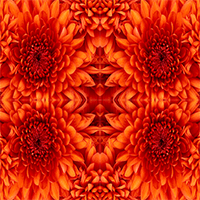I needed a tiled background for something I was working on and decided to revisit my mirror program. I’ve lost the source code to my original program from 2005, but it wasn’t too hard to rewrite it.

I decided to implement this one as an extension method on the Bitmap class. Here is the code for the extension:

``````namespace MirrorMirror
{
public static class Utilities {
public static Bitmap CreateMirrorImage(this Bitmap srcImage)
{
int dblWidth = srcImage.Width * 2;
int dblHeight = srcImage.Height * 2; // create an image 2 times the size of the original
Bitmap newImage = new Bitmap(dblWidth, dblHeight);
using (Graphics canvas = Graphics.FromImage(newImage))
{
// place the original image in the top left corner
canvas.DrawImage(srcImage, new Point { X = 0, Y = 0 });

// rotate and flip the image to create a mirror
srcImage.RotateFlip(RotateFlipType.RotateNoneFlipX);
canvas.DrawImage(srcImage, new Point { X = srcImage.Width, Y = 0 });
srcImage.RotateFlip(RotateFlipType.Rotate180FlipNone);
canvas.DrawImage(srcImage, new Point { X = 0, Y = srcImage.Height});
srcImage.RotateFlip(RotateFlipType.RotateNoneFlipX);
canvas.DrawImage(srcImage, new Point { X = srcImage.Width, Y = srcImage.Height });
canvas.Save();
}
return newImage;
}
}
}``````

To use this code, simply do the following:

``````openFileDialog1.ShowDialog();
Bitmap srcImage = new Bitmap(openFileDialog1.FileName);
pictureBox1.Image = srcImage.CreateMirrorImage();``````

As you can see, you’ll need a picture box and an open file dialog on the form for the sample code to work.

This is much simpler than my original program. Here are a few samples of what you can create using it…

Here is the original image:Here is the first pass of the tile:And the second pass of the tile: